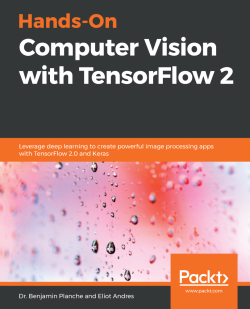### Hands-On Computer Vision with TensorFlow 23 (1 reviews total)
By Benjamin Planche , Eliot Andres
• FREE Subscribe Access now
• \$34.99 Print + eBook
• \$27.99 eBook
• Constantly updated with 100+ new titles each month
• Breadth and depth in over 1,000+ technologies

Computer vision solutions are becoming increasingly common, making their way into fields such as health, automobile, social media, and robotics. This book will help you explore TensorFlow 2, the brand new version of Google's open source framework for machine learning. You will understand how to benefit from using convolutional neural networks (CNNs) for visual tasks.

Hands-On Computer Vision with TensorFlow 2 starts with the fundamentals of computer vision and deep learning, teaching you how to build a neural network from scratch. You will discover the features that have made TensorFlow the most widely used AI library, along with its intuitive Keras interface. You'll then move on to building, training, and deploying CNNs efficiently. Complete with concrete code examples, the book demonstrates how to classify images with modern solutions, such as Inception and ResNet, and extract specific content using You Only Look Once (YOLO), Mask R-CNN, and U-Net. You will also build generative adversarial networks (GANs) and variational autoencoders (VAEs) to create and edit images, and long short-term memory networks (LSTMs) to analyze videos. In the process, you will acquire advanced insights into transfer learning, data augmentation, domain adaptation, and mobile and web deployment, among other key concepts.

By the end of the book, you will have both the theoretical understanding and practical skills to solve advanced computer vision problems with TensorFlow 2.0.

Publication date:
May 2019
Publisher
Packt
Pages
372
ISBN
9781788830645

# Computer Vision and Neural Networks

In recent years, computer vision has grown into a key domain for innovation, with more and more applications reshaping businesses and lifestyles. We will start this book with a brief presentation of this field and its history so that we can get some background information. We will then introduce artificial neural networks and explain how they have revolutionized computer vision. Since we believe in learning through practice, by the end of this first chapter, we will even have implemented our own network from scratch!

The following topics will be covered in this chapter:

• Computer vision and why it is a fascinating contemporary domain
• How we got there—from local hand-crafted descriptors to deep neural networks
• Neural networks, what they actually are, and how to implement our own for a basic recognition task

# Technical requirements

Throughout this book, we will be using Python 3.5 (or higher). As a general-purpose programming language, Python has become the main tool for data scientists thanks to its useful built-in features and renowned libraries.

For this introductory chapter, we will only use two cornerstone libraries—NumPy and Matplotlib. They can be found at and installed from www.numpy.org and matplotlib.org. However, we recommend using Anaconda (www.anaconda.com), a free Python distribution that makes package management and deployment easy.

Complete installation instructions—as well as all the code presented alongside this chapter—can be found in the GitHub repository at github.com/PacktPublishing/Hands-On-Computer-Vision-with-TensorFlow2/tree/master/Chapter01.

We assume that our readers already have some knowledge of Python and a basic understanding of image representation (pixels, channels, and so on) and matrix manipulation (shapes, products, and so on).

# Computer vision in the wild

Computer vision is everywhere nowadays, to the point that its definition can drastically vary from one expert to another. In this introductory section, we will paint a global picture of computer vision, highlighting its domains of application and the challenges it faces.

# Introducing computer vision

Computer vision can be hard to define because it sits at the junction of several research and development fields, such as computer science (algorithms, data processing, and graphics), physics (optics and sensors), mathematics (calculus and information theory), and biology (visual stimuli and neural processing). At its core, computer vision can be summarized as the automated extraction of information from digital images.

Our brain works wonders when it comes to vision. Our ability to decipher the visual stimuli our eyes constantly capture, to instantly tell one object from another, and to recognize the face of someone we have met only once, is just incredible. For computers, images are just blobs of pixels, matrices of red-green-blue values with no further meaning.

The goal of computer vision is to teach computers how to make sense of these pixels the way humans (and other creatures) do, or even better. Indeed, computer vision has come a long way and, since the rise of deep learning, it has started achieving super human performance in some tasks, such as face verification and handwritten text recognition.

With a hyper active research community fueled by the biggest IT companies, and the ever-increasing availability of data and visual sensors, more and more ambitious problems are being tackled: vision-based navigation for autonomous driving, content-based image and video retrieval, and automated annotation and enhancement, among others. It is truly an exciting time for experts and newcomers alike.

# Main tasks and their applications

New computer vision-based products are appearing every day (for instance, control systems for industries, interactive smartphone apps, and surveillance systems) that cover a wide range of tasks. In this section, we will go through the main ones, detailing their applications in relation to real-life problems.

# Content recognition

A central goal in computer vision is to make sense of images, that is, to extract meaningful, semantic information from pixels (such as the objects present in images, their location, and their number). This generic problem can be divided into several sub-domains. Here is a non-exhaustive list.

# Object classification

Object classification (or image classification) is the task of assigning proper labels (or classes) to images among a predefined set and is illustrated in the following diagram: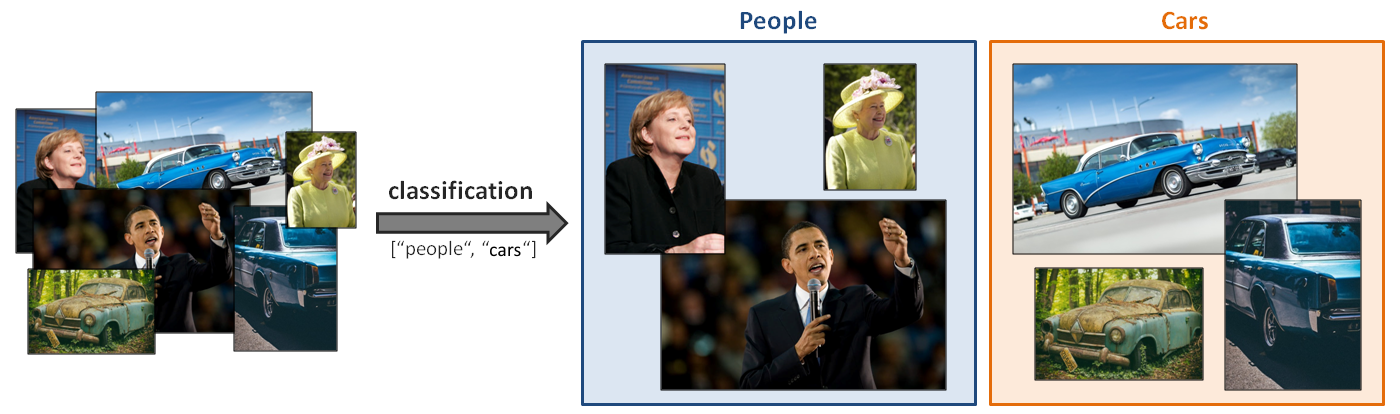Figure 1.1: Example of a classifier for the labels of people and cars applied to an image set

Object classification became famous for being the first success story of deep convolutional neural networks being applied to computer vision back in 2012 (this will be presented later in this chapter). Progress in this domain has been so fast since then that super human performance is now achieved in various use cases (a well-known example is the classification of dog breeds; deep learning methods have become extremely efficient at spotting the discriminative features of man's best friend).

Common applications are text digitization (using character recognition) and the automatic annotation of image databases.

In Chapter 4, Influential Classification Tools, we will present advanced classification methods and their impact on computer vision in general.

# Object identification

While object classification methods assign labels from a predefined set, object identification (or instance classification) methods learn to recognize specific instances of a class.

For example, an object classification tool could be configured to return images containing faces, while an identification method would focus on the face's features to identify the person and recognize them in other images (identifying each face in all of the images, as shown in the following diagram):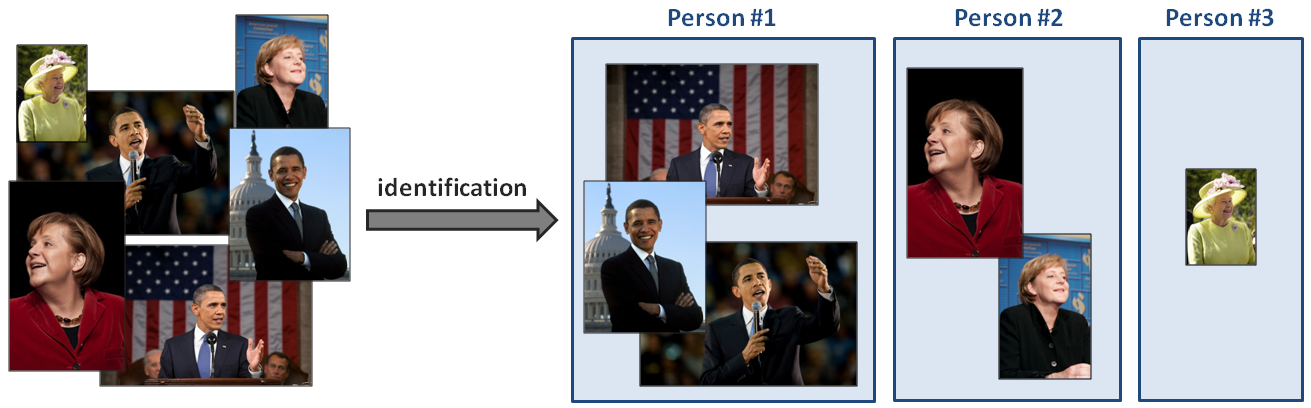Figure 1.2: Example of an identifier applied to portraits

Therefore, object identification can be seen as a procedure to cluster a dataset, often applying some dataset analysis concepts (which will be presented in Chapter 6, Enhancing and Segmenting Images).

# Object detection and localization

Another task is the detection of specific elements in an image. It is commonly applied to face detection for surveillance applications or even advanced camera apps, the detection of cancerous cells in medicine, the detection of damaged components in industrial plants, and so on.

Detection is often a preliminary step before further computations, providing smaller patches of the image to be analyzed separately (for instance, cropping someone's face for facial recognition, or providing a bounding box around an object to evaluate its pose for augmented reality applications), as shown in the following diagram: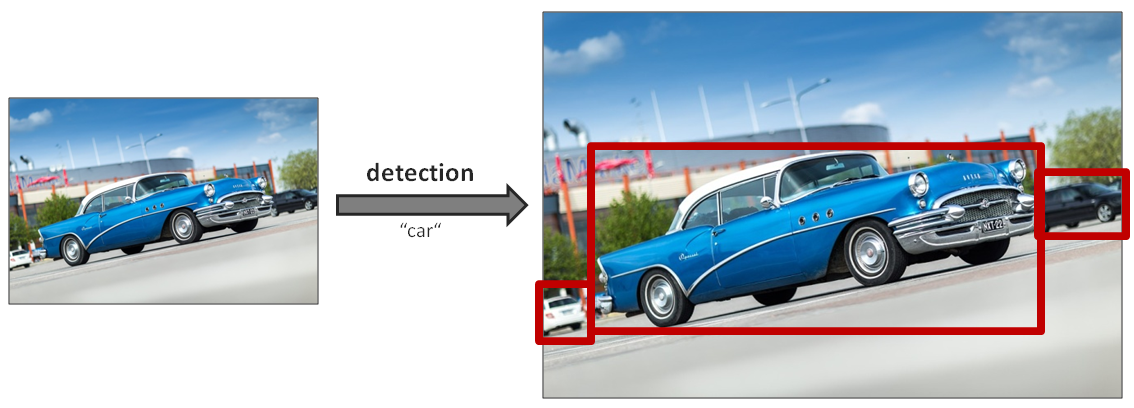Figure 1.3: Example of a car detector, returning bounding boxes for the candidates

State-of-the-art solutions will be detailed in Chapter 5, Object Detection Models.

# Object and instance segmentation

Segmentation can be seen as a more advanced type of detection. Instead of simply providing bounding boxes for the recognized elements, segmentation methods return masks labeling all the pixels belonging to a specific class or to a specific instance of a class (refer to the following Figure 1.4). This makes the task much more complex, and actually one of the few in computer vision where deep neural networks are still far from human performance (our brain is indeed remarkably efficient at drawing the precise boundaries/contours of visual elements). Object segmentation and instance segmentation are illustrated in the following diagram: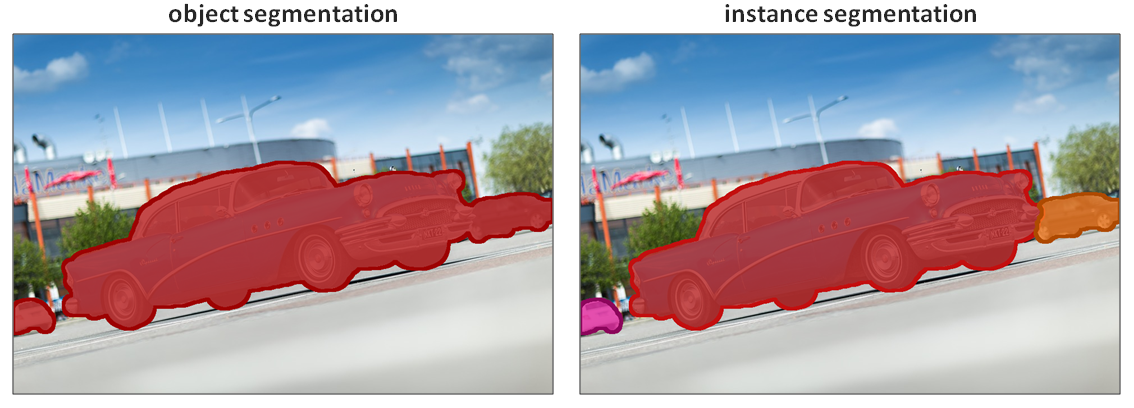Figure 1.4: Comparing the results of object segmentation methods and instance segmentation methods for cars

In Figure 1.4, while the object segmentation algorithm returns a single mask for all pixels belonging to the car class, the instance segmentation one returns a different mask for each car instance that it recognized. This is a key task for robots and smart cars in order to understand their surroundings (for example, to identify all the elements in front of a vehicle), but it is also used in medical imagery. Precisely segmenting the different tissues in medical scans can enable faster diagnosis and easier visualization (such as coloring each organ differently or removing clutter from the view). This will be demonstrated in Chapter 6, Enhancing and Segmenting Images, with concrete experiments for autonomous driving applications.

# Pose estimation

Pose estimation can have different meanings depending on the targeted tasks. For rigid objects, it usually means the estimation of the objects' positions and orientations relative to the camera in the 3D space. This is especially useful for robots so that they can interact with their environment (object picking, collision avoidance, and so on). It is also often used in augmented reality to overlay 3D information on top of objects.

For non-rigid elements, pose estimation can also mean the estimation of the positions of their sub-parts relative to each other. More concretely, when considering humans as non-rigid targets, typical applications are the recognition of human poses (standing, sitting, running, and so on) or understanding sign language. These different cases are illustrated in the following diagram: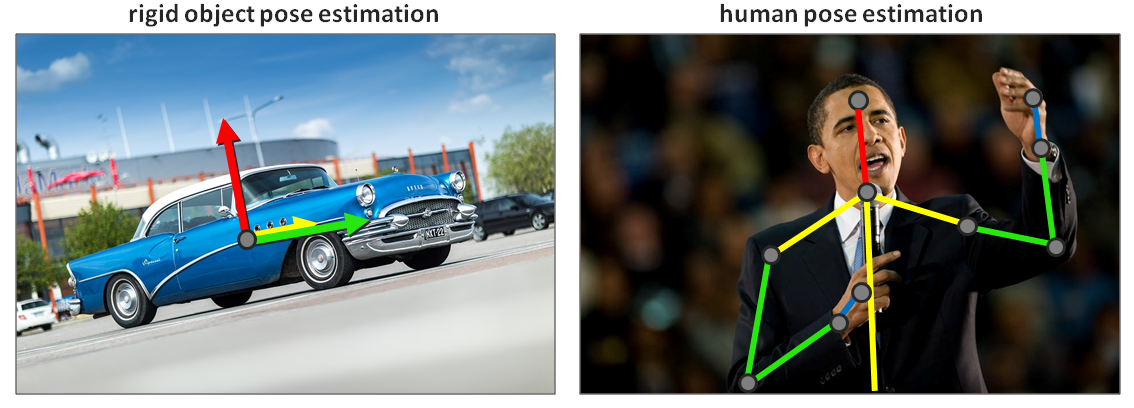Figure 1.5: Examples of rigid and non-rigid pose estimation

In both cases—that is, for whole or partial elements—the algorithms are tasked with evaluating their actual position and orientation relative to the camera in the 3D world, based on their 2D representation in an image.

# Video analysis

Computer vision not only applies to single images, but also to videos. If video streams are sometimes analyzed frame by frame, some tasks require that you consider an image sequence as a whole in order to take temporal consistency into account (this will be one of the topics of Chapter 8, Video and Recurrent Neural Networks).

# Instance tracking

Some tasks relating video streams could naively be accomplished by studying each frame separately (memory less), but more efficient methods either take into account differences from image to image to guide the process to new frames or take complete image sequences as input for their predictions. Tracking, that is, localizing specific elements in a video stream, is a good example of such a task.

Tracking could be done frame by frame by applying detection and identification methods to each frame. However, it is much more efficient to use previous results to model the motion of the instances in order to partially predict their locations in future frames. Motion continuity is, therefore, a key predicate here, though it does not always hold (such as for fast-moving objects).

# Action recognition

On the other hand, action recognition belongs to the list of tasks that can only be run with a sequence of images. Similar to how we cannot understand a sentence when we are given the words separately and unordered, we cannot recognize an action without studying a continuous sequence of images (refer to Figure 1.6).

Recognizing an action means recognizing a particular motion among a predefined set (for instance, for human actions—dancing, swimming, drawing a square, or drawing a circle). Applications range from surveillance (such as the detection of abnormal or suspicious behavior) to human-machine interactions (such as for gesture-controlled devices):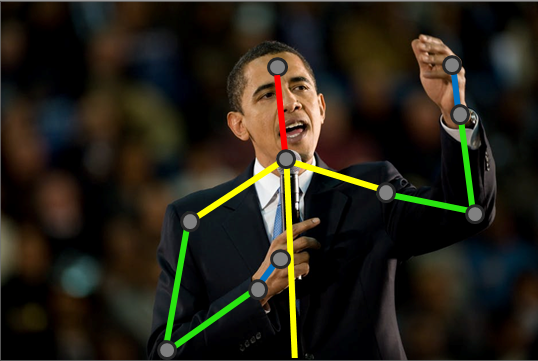Figure 1.6: Is Barack Obama in the middle of waving, pointing at someone, swatting a mosquito, or something else?
Only the complete sequence of frames could help to label this action
Since object recognition can be split into object classification, detection, segmentation, and so on, so can action recognition (action classification, detection, and so on).

# Motion estimation

Instead of trying to recognize moving elements, some methods focus on estimating the actual velocity/trajectory that is captured in videos. It is also common to evaluate the motion of the camera itself relative to the represented scene (egomotion). This is particularly useful in the entertainment industry, for example, to capture motion in order to apply visual effects or to overlay 3D information in TV streams such as sports broadcasting.

# Content-aware image edition

Besides the analysis of their content, computer vision methods can also be applied to improve the images themselves. More and more, basic image processing tools (such as low-pass filters for image denoising) are being replaced by smarter methods that are able to use prior knowledge of the image content to improve its visual quality. For instance, if a method learns what a bird typically looks like, it can apply this knowledge in order to replace noisy pixels with coherent ones in bird pictures. This concept applies to any type of image restoration, whether it be denoising, deblurring, or resolution enhancing (super-resolution, as illustrated in the following diagram):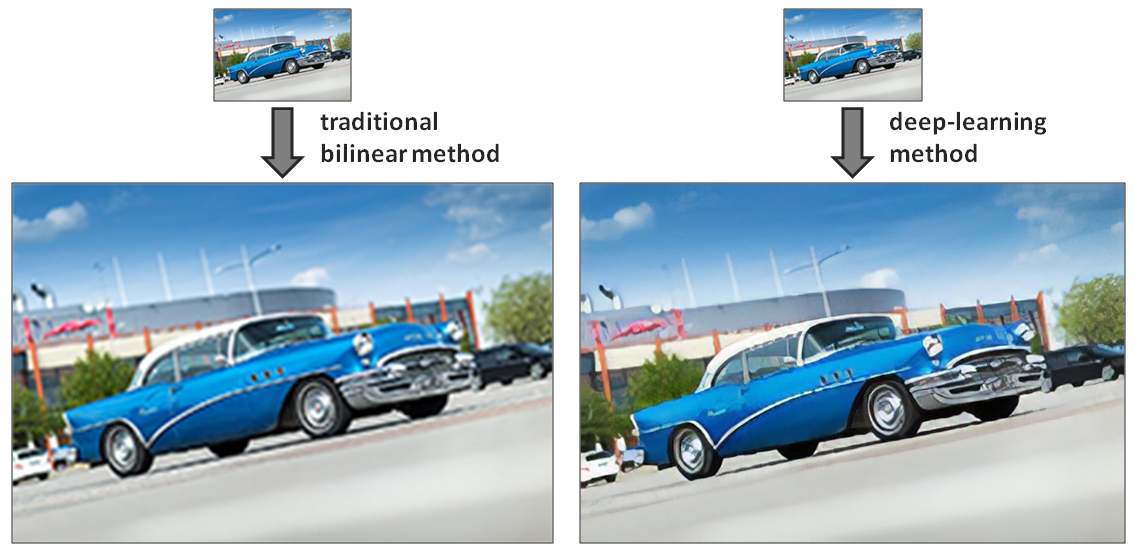Figure 1.7: Comparison of traditional and deep learning methods for image super-resolution. Notice how the details are sharper in the second image

Content-aware algorithms are also used in some photography or art applications, such as the smart portrait or beauty modes for smartphones, which aim to enhance some of the models' features, or the smart removing/editing tools, which get rid of unwanted elements and replace them with a coherent background.

In Chapter 6, Enhancing and Segmenting Images, and in Chapter 7, Training on Complex and Scarce Datasets, we will demonstrate how such generative methods can be built and served.

# Scene reconstruction

Finally, though we won't tackle it in this book, scene reconstruction is the task of recovering the 3D geometry of a scene, given one or more images. A simple example, based on human vision, is stereo matching. This is the process of finding correspondences between two images of a scene from different viewpoints in order to derive the distance of each visualized element. More advanced methods take several images and match their content together in order to obtain a 3D model of the target scene. This can be applied to the 3D scanning of objects, people, buildings, and so on.

# A brief history of computer vision

"Study the past if you would define the future."
– Confucius

In order to better understand the current stand of the heart and current challenges of computer vision, we suggest that we quickly have a look at where it came from and how it has evolved in the past decades.

# First steps to initial successes

Scientists have long dreamed of developing artificial intelligence, including visual intelligence. The first advances in computer vision were driven by this idea.

Computer vision as a domain started as early as the 60s, among the Artificial Intelligence (AI) research community. Still heavily influenced by the symbolist philosophy, which considered playing chess and other purely intellectual activities the epitome of human intelligence, these researchers underestimated the complexity of lower animal functions such as perception. How these researchers believed they could reproduce human perception through a single summer project in 1966 is a famous anecdote in the computer vision community.

Marvin Minsky was one of the first to outline an approach toward building AI systems based on perception (in Steps toward artificial intelligence, Proceedings of the IRE, 1961). He argued that with the use of lower functions such as pattern recognition, learning, planning, and induction, it could be possible to build machines capable of solving a broad variety of problems. However, this theory was only properly explored from the 80s onward. In Locomotion, Vision, and Intelligence in 1984, Hans Moravec noted that our nervous system, through the process of evolution, has developed to tackle perceptual tasks (more than 30% of our brain is dedicated to vision!).

As he noted, even if computers are pretty good at arithmetic, they cannot compete with our perceptual abilities. In this sense, programming a computer to solve purely intellectual tasks (for example, playing chess) does not necessarily contribute to the development of systems that are intelligent in a general sense or relative to human intelligence.

# Hand-crafting local features

Inspired by human perception, the basic mechanisms of computer vision are straightforward and have not evolved much since the early years—the idea is to first extract meaningful features from the raw pixels, and then match these features to known, labeled ones in order to achieve recognition.

In computer vision, a feature is a piece of information (often mathematically represented as a one or two-dimensional vector) that is extracted from data that is relevant to the task at hand. Features include some key points in the images, specific edges, discriminative patches, and so on. They should be easy to obtain from new images and contain the necessary information for further recognition.

Researchers used to come up with more and more complex features. The extraction of edges and lines was first considered for the basic geometrical understanding of scenes or for character recognition; then, texture and lighting information was also taken into account, leading to early object classifiers.

In the 90s, features based on statistical analysis, such as principal component analysis (PCA), were successfully applied for the first time to complex recognition problems such as face classification. A classic example is the Eigenface method introduced by Matthew Turk and Alex Pentland (Eigenfaces for Recognition, MIT Press, 1991). Given a database of face images, the mean image and the eigenvectors/images (also known as characteristic vectors/images) were computed through PCA. This small set of eigenimages can theoretically be linearly combined to reconstruct any face in the original dataset, or beyond. In other words, each face picture can be approximated through a weighted sum of the eigenimages (refer to Figure 1.8). This means that a particular face can simply be defined by the list of reconstruction weights for each eigenimage. As a result, classifying a new face is just a matter of decomposing it into eigenimages to obtain its weight vector, and then comparing it with the vectors of known faces: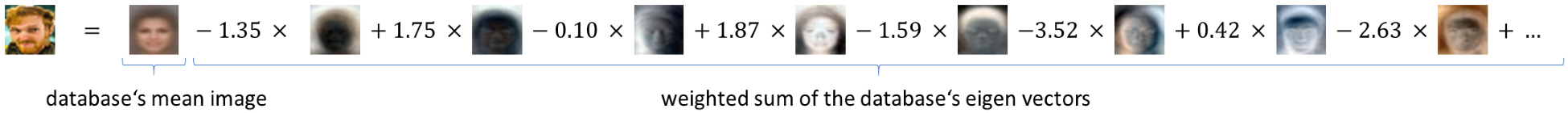Figure 1.8: Decomposition of a portrait image into the mean image and weighted sum of eigenimages. These mean and eigenimages were computed over a larger face dataset

Another method that appeared in the late 90s and revolutionized the domain is called Scale Invariant Feature Transform (SIFT). As its name suggests, this method, introduced by David Lowe (in Distinctive Image Features from Scale-Invariant Keypoints, Elsevier), represents visual objects by a set of features that are robust to changes in scale and orientation. In the simplest terms, this method looks for some key points in images (searching for discontinuities in their gradient), extracts a patch around each key point, and computes a feature vector for each (for example, a histogram of the values in the patch or in its gradient). The local features of an image, along with their corresponding key points, can then be used to match similar visual elements across other images. In the following image, the SIFT method was applied to a picture using OpenCV (https://docs.opencv.org/3.1.0/da/df5/tutorial_py_sift_intro.html). For each localized key point, the radius of the circle represents the size of the patch considered for the feature computation, and the line shows the feature orientation (that is, the main orientation of the neighborhood's gradient):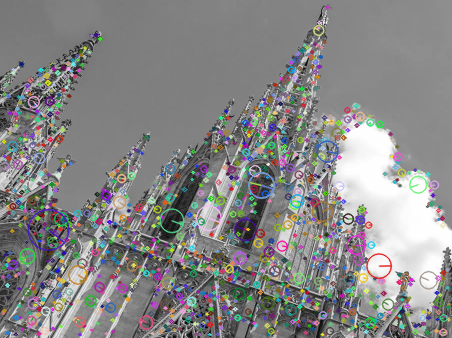Figure 1.9: Representation of the SIFT key points extracted from an image (using OpenCV)

More advanced methods were developed over the years—with more robust ways of extracting key points, or computing and combining discriminative features—but they followed the same overall procedure (extracting features from one image, and comparing them to the features of others).

# Adding some machine learning on top

It soon appeared clear, however, that extracting robust, discriminative features was only half the job for recognition tasks. For instance, different elements from the same class can look quite different (such as different-looking dogs) and, as a result, share only a small set of common features. Therefore, unlike image-matching tasks, higher-level problems such as semantic classification cannot be solved by simply comparing pixel features from query images with those from labeled pictures (such a procedure can also become sub-optimal in terms of processing time if the comparison has to be done with every image from a large labeled dataset).

This is where machine learning come into play. With an increasing number of researchers trying to tackle image classification in the 90s, more statistical ways to discriminate images based on their features started to appear. Support vector machines (SVMs), which were standardized by Vladimir Vapnik and Corinna Cortes (Support-vector networks, Springer, 1995), were, for a long time, the default solution for learning a mapping from complex structures (such as images) to simpler labels (such as classes).

Given a set of image features and their binary labels (for example, cat or not cat, as illustrated in Figure 1.10), an SVM can be optimized to learn the function to separate one class from another, based on extracted features. Once this function is obtained, it is just a matter of applying it to the feature vector of an unknown image so that we can map it to one of the two classes (SVMs that could extend to a larger number of classes were later developed). In the following diagram, an SVM was taught to regress a linear function separating two classes based on features extracted from their images (features as vectors of only two values in this example):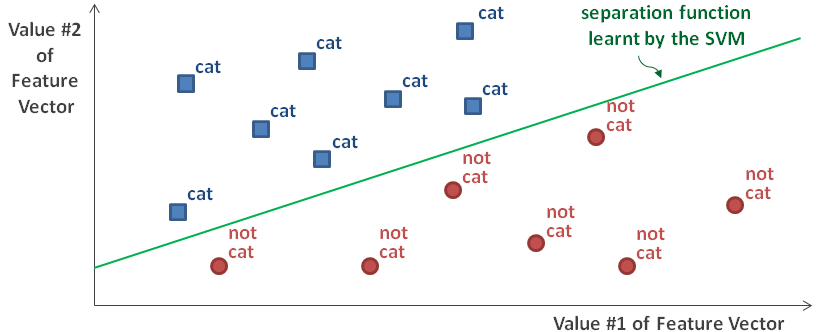Figure 1.10: An illustration of a linear function regressed by an SVM. Note that using a concept known as the kernel trick, SVMs can also find non-linear solutions to separate classes

Other machine learning algorithms were adapted over the years by the computer vision community, such as random forests, bags of words, Bayesian models, and obviously neural networks.

# Rise of deep learning

So, how did neural networks take over computer vision and become what we nowadays know as deep learning? This section offers some answers, detailing the technical development of this powerful tool.

# Early attempts and failures

It may be surprising to learn that artificial neural networks appeared even before modern computer vision. Their development is the typical story of an invention too early for its time.

# Rise and fall of the perceptron

In the 50s, Frank Rosenblatt came up with the perceptron, a machine learning algorithm inspired by neurons and the underlying block of the first neural networks (The Perceptron: A Probabilistic Model for Information Storage and Organization in the Brain, American Psychological Association, 1958). With the proper learning procedure, this method was already able to recognize characters. However, the hype was short-lived. Marvin Minsky (one of the fathers of AI) and Seymor Papert quickly demonstrated that the perceptron could not learn a function as simple as XOR (exclusive OR, the function that, given two binary input values, returns 1 if one, and only one, input is 1, and returns 0 otherwise). This makes sense to us nowadays—as the perceptron back then was modeled with a linear function while XOR is a non-linear one—but, at that time, it simply discouraged any further research for years.

# Too heavy to scale

It was only in the late 70s to early 80s that neural networks got some attention put back on them. Several research papers introduced how neural networks, with multiple layers of perceptrons put one after the other, could be trained using a rather straightforward scheme—backpropagation. As we will detail in the next section, this training procedure works by computing the network's error and backpropagating it through the layers of perceptrons to update their parameters using derivatives. Soon after, the first convolutional neural network (CNN), the ancestor of current recognition methods, was developed and applied to the recognition of handwritten characters with some success.

Alas, these methods were computationally heavy, and just could not scale to larger problems. Instead, researchers adopted lighter machine learning methods such as SVMs, and the use of neural networks stalled for another decade. So, what brought them back and led to the deep learning era we know of today?

# Reasons for the comeback

The reasons for this comeback are twofold and rooted in the explosive evolution of the internet and hardware efficiency.

# The internet – the new El Dorado of data science

The internet was not only a revolution in communication; it also deeply transformed data science. It became much easier for scientists to share images and content by uploading them online, leading to the creation of public datasets for experimentation and benchmarking. Moreover, not only researchers but soon everyone, all over the world, started adding new content online, sharing images, videos, and more at an exponential rate. This started big data and the golden age of data science, with the internet as the new El Dorado.

By simply indexing the content that is constantly published online, image and video datasets reached sizes that were never imagined before, from Caltech-101 (10,000 images, published in 2003 by Li Fei-Fei et al., Elsevier) to ImageNet (14+ million images, published in 2009 by Jia Deng et al., IEEE) or Youtube-8M (8+ million videos, published in 2016 by Sami Abu-El-Haija et al., including Google). Even companies and governments soon understood the numerous advantages of gathering and releasing datasets to boost innovation in their specific domains (for example, the i-LIDS datasets for video surveillance released by the British government and the COCO dataset for image captioning sponsored by Facebook and Microsoft, among others).

With so much data available covering so many use cases, new doors were opened (data-hungry algorithms, that is, methods requiring a lot of training samples to converge could finally be applied with success), and new challenges were raised (such as how to efficiently process all this information).

# More power than ever

Luckily, since the internet was booming, so was computing power. Hardware kept becoming cheaper as well as faster, seemingly following Moore's famous law (which states that processor speeds should double every two years—this has been true for almost four decades, though a deceleration is now being observed). As computers got faster, they also became better designed for computer vision. And for this, we have to thank video games.

The graphical processing unit (GPU) is a computer component, that is, a chip specifically designed to handle the kind of operations needed to run 3D games. Therefore, a GPU is optimized to generate or manipulate images, parallelizing these heavy matrix operations. Though the first GPUs were conceived in the 80s, they became affordable and popular only with the advent of the new millennium.

In 2007, NVIDIA, one of the main companies designing GPUs, released the first version of CUDA, a programming language that allows developers to directly program for compatible GPUs. OpenCL, a similar language, appeared soon after. With these new tools, people started to harness the power of GPUs for new tasks, such as machine learning and computer vision.

# Deep learning or the rebranding of artificial neural networks

The conditions were finally there for data-hungry, computationally-intensive algorithms to shine. Along with big data and cloud computing, deep learning was suddenly everywhere.

# What makes learning deep?

Actually, the term deep learning had already been coined back in the 80s, when neural networks first began stacking two or three layers of neurons. As opposed to the early, simpler solutions, deep learning regroups deeper neural networks, that is, networks with multiple hidden layers—additional layers set between their input and output layers. Each layer processes its inputs and passes the results to the next layer, all trained to extract increasingly abstract information. For instance, the first layer of a neural network would learn to react to basic features in the images, such as edges, lines, or color gradients; the next layer would learn to use these cues to extract more advanced features; and so on until the last layer, which infers the desired output (such as predicted class or detection results).

However, deep learning only really started being used from 2006, when Geoff Hinton and his colleagues proposed an effective solution to train these deeper models, one layer at a time, until reaching the desired depth (A Fast Learning Algorithm for Deep Belief Nets, MIT Press, 2006).

# Deep learning era

With research into neural networks once again back on track, deep learning started growing, until a major breakthrough in 2012, which finally gave it its contemporary prominence. Since the publication of ImageNet, a competition (ImageNet Large Scale Visual Recognition Challenge (ILSVRC)—image-net.org/challenges/LSVRC) has been organized every year for researchers to submit their latest classification algorithms and compare their performance on ImageNet with others. The winning solutions in 2010 and 2011 had classification errors of 28% and 26% respectively, and applied traditional concepts such as SIFT features and SVMs. Then came the 2012 edition, and a new team of researchers reduced the recognition error to a staggering 16%, leaving all the other contestants far behind.

In their paper describing this achievement (Imagenet Classification with Deep Convolutional Neural Networks, NIPS, 2012), Alex Krizhevsky, Ilya Sutskever, and Geoff Hinton presented what would become the basis for modern recognition methods. They conceived an 8-layer neural network, later named AlexNet, with several convolutional layers and other modern components such as dropout and rectified linear activation units (ReLUs), which will all be presented in detail in Chapter 3, Modern Neural Networks, as they have became central to computer vision. More importantly, they used CUDA to implement their method so that it can be run on GPUs, finally making it possible to train deep neural networks in a reasonable time, iterating over datasets as big as ImageNet.

That same year, Google demonstrated how advances in cloud computing could also be applied to computer vision. Using a dataset of 10 million random images extracted from YouTube videos, they taught a neural network to identify images containing cats and parallelized the training process over 16,000 machines to finally double the accuracy compared to previous methods.

And so started the deep learning era we are currently in. Everyone jumped on board, coming up with deeper and deeper models, more advanced training schemes, and lighter solutions for portable devices. It is an exciting period, as the more efficient deep learning solutions become, the more people try to apply them to new applications and domains. With this book, we hope to convey some of this current enthusiasm and provide you with an overview of the modern methods and how to develop solutions.

# Getting started with neural networks

By now, we know that neural networks form the core of deep learning and are powerful tools for modern computer vision. But what are they exactly? How do they work? In the following section, not only will we tackle the theoretical explanations behind their efficiency, but we will also directly apply this knowledge to the implementation and application of a simple network to a recognition task.

# Building a neural network

Artificial neural networks (ANNs), or simply neural networks (NNs), are powerful machine learning tools that are excellent at processing information, recognizing usual patterns or detecting new ones, and approximating complex processes. They have to thank their structure for this, which we will now explore.

# Imitating neurons

It is well-known that neurons are the elemental supports of our thoughts and reactions. What might be less evident is how they actually work and how they can be simulated.

# Biological inspiration

ANNs are loosely inspired by how animals' brains work. Our brain is a complex network of neurons, each passing information to each other and processing sensory inputs (as electrical and chemical signals) into thoughts and actions. Each neuron receives its electrical inputs from its dendrites, which are cell fibers that propagate the electrical signal from the synapses (the junctions with preceding neurons) to the soma (the neuron's main body). If the accumulated electrical stimulation exceeds a specific threshold, the cell is activated and the electrical impulse is propagated further to the next neurons through the cell's axon (the neuron's output cable, ending with several synapses linking to other neurons). Each neuron can, therefore, be seen as a really simple signal processing unit, which—once stacked together—can achieve the thoughts we are having right now, for instance.

# Mathematical model

Inspired by its biological counterpart (represented in Figure 1.11), the artificial neuron takes several inputs (each a number), sums them together, and finally applies an activation function to obtain the output signal, which can be passed to the following neurons in the network (this can be seen as a directed graph):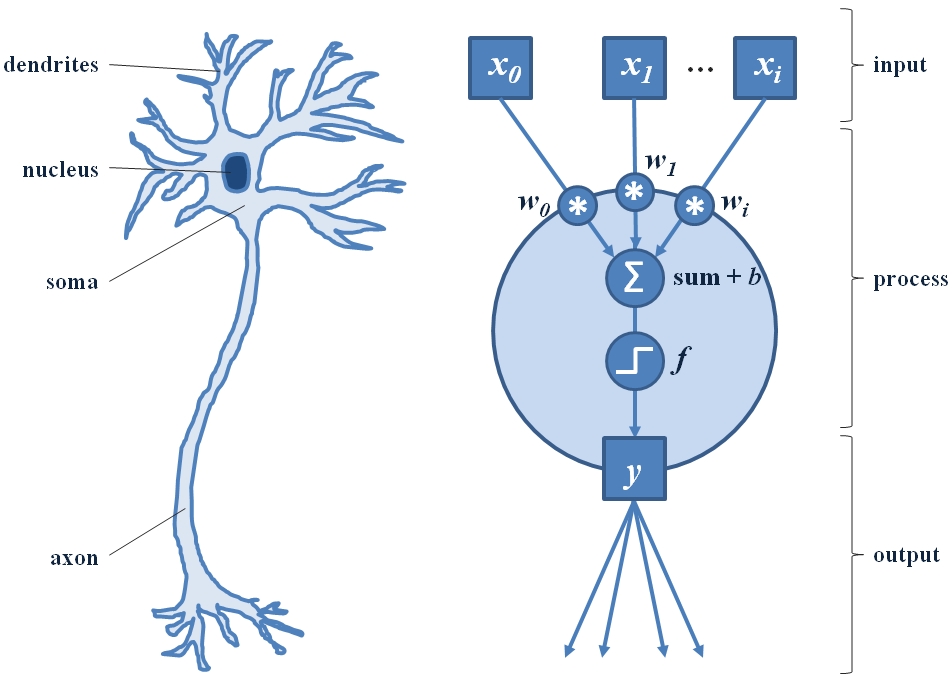Figure 1.11: On the left, we can see a simplified biological neuron. On the right, we can see its artificial counterpart

The summation of the inputs is usually done in a weighted way. Each input is scaled up or down, depending on a weight specific to this particular input. These weights are the parameters that are adjusted during the training phase of the network in order for the neuron to react to the correct features. Often, another parameter is also trained and used for this summation process—the neuron's bias. Its value is simply added to the weighted sum as an offset.

Let's quickly formalize this process mathematically. Suppose we have a neuron that takes two input values, x0 and x1. Each of these values would be weighted by a factor, w0 and w1, respectively, before being summed together, with an optional bias, b. For simplification, we can express the input values as a horizontal vector, x, and the weights as a vertical vector, w:With this formulation, the whole operation can simply be expressed as follows:This step is straightforward, isn't it? The dot product between the two vectors takes care of the weighted summation:Now that the inputs have been scaled and summed together into the result, z, we have to apply the activation function to it in order to get the neuron's output. If we go back to the analogy with the biological neuron, its activation function would be a binary function such as if y is above a threshold t, return an electrical impulse that is 1, or else return 0 (with t = 0 usually). If we formalize this, the activation function, y = f(z), can be expressed as follows:The step function is a key component of the original perceptron, but more advanced activation functions have been introduced since then with more advantageous properties, such as non-linearity (to model more complex behaviors) and continuous differentiability (important for the training process, which we will explain later). The most common activation functions are as follows:

• The sigmoid function,(with 𝑒 the exponential function)
• The hyperbolic tangent,• The REctified Linear Unit (ReLU),Plots of the aforementioned common activation functions are shown in the following diagram: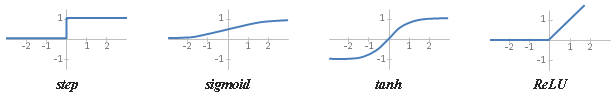Figure 1.12: Plotting common activation functions

In any case, that's it! We have modeled a simple artificial neuron. It is able to receive a signal, process it, and output a value that can be forwarded (a term that is commonly used in machine learning) to other neurons, building a network.

Chaining neurons with no non-linear activation functions would be equivalent to having a single neuron. For instance, if we had a linear neuron with parameters wA and bA followed by a linear neuron with parameters wB and bB, then, where wwA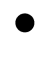wB and b = bA + bB. Therefore, non-linear activation functions are a necessity if we want to create complex models.

# Implementation

Such a model can be implemented really easily in Python (using NumPy for vector and matrix manipulations):

`import numpy as npclass Neuron(object):    """A simple feed-forward artificial neuron.    Args:        num_inputs (int): The input vector size / number of input values.        activation_fn (callable): The activation function.    Attributes:        W (ndarray): The weight values for each input.        b (float): The bias value, added to the weighted sum.        activation_fn (callable): The activation function.    """    def __init__(self, num_inputs, activation_fn):        super().__init__()        # Randomly initializing the weight vector and bias value:        self.W = np.random.rand(num_inputs)        self.b = np.random.rand(1)        self.activation_fn = activation_fn    def forward(self, x):        """Forward the input signal through the neuron."""        z = np.dot(x, self.W) + self.b        return self.activation_function(z)`

As we can see, this is a direct adaptation of the mathematical model we defined previously. Using this artificial neuron is just as straightforward. Let's instantiate a perceptron (a neuron with the step function for the activation method) and forward a random input through it:

`# Fixing the random number generator's seed, for reproducible results:np.random.seed(42)# Random input column array of 3 values (shape = `(1, 3)`)x = np.random.rand(3).reshape(1, 3)# > [[0.37454012 0.95071431 0.73199394]]# Instantiating a Perceptron (simple neuron with step function):step_fn = lambda y: 0 if y <= 0 else 1perceptron = Neuron(num_inputs=x.size, activation_fn=step_fn)# > perceptron.weights    = [0.59865848 0.15601864 0.15599452]# > perceptron.bias       = [0.05808361]out = perceptron.forward(x)# > 1`

We suggest that you take some time and experiment with different inputs and neuron parameters before we scale up their dimensions in the next section.

# Layering neurons together

Usually, neural networks are organized into layers, that is, sets of neurons that typically receive the same input and apply the same operation (for example, by applying the same activation function, though each neuron first sums the inputs with its own specific weights).

# Mathematical model

In networks, the information flows from the input layer to the output layer, with one or more hidden layers in-between. In Figure 1.13, the three neurons A, B, and C belong to the input layer, the neuron H belongs to the output or activation layer, and the neurons D, E, F, and G belong to the hidden layer. The first layer has an input, x, of size 2, the second (hidden) layer takes the three activation values of the previous layer as input, and so on. Such layers, with each neuron connected to all the values from the previous layer, are classed as being fully connected or dense: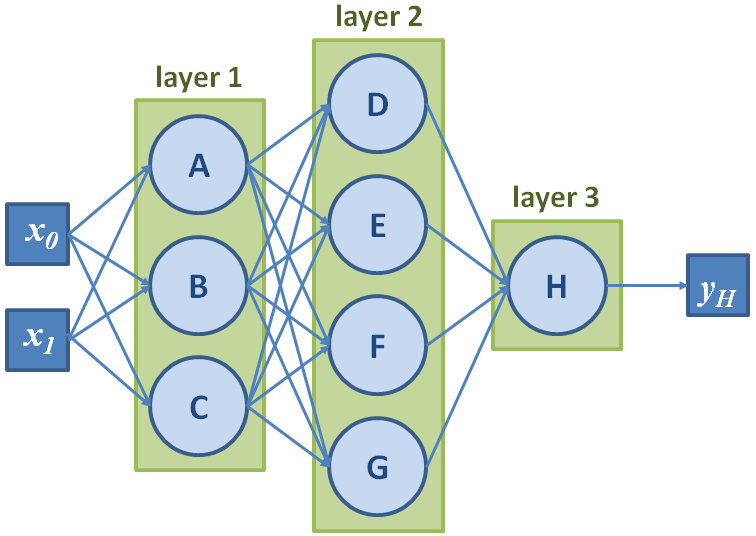Figure 1.13: A 3-layer neural network, with two input values and one final output

Once again, we can compact the calculations by representing these elements with vectors and matrices. The following operations are done by the first layers: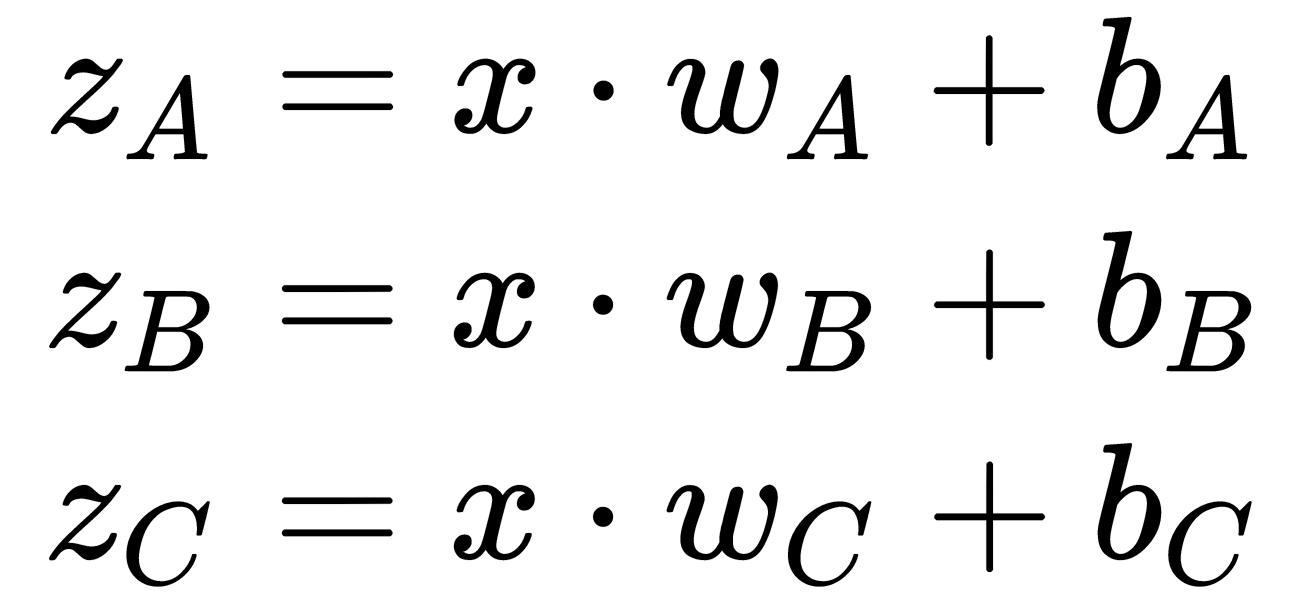This can be expressed as follows:In order to obtain the previous equation, we must define the variables as follows: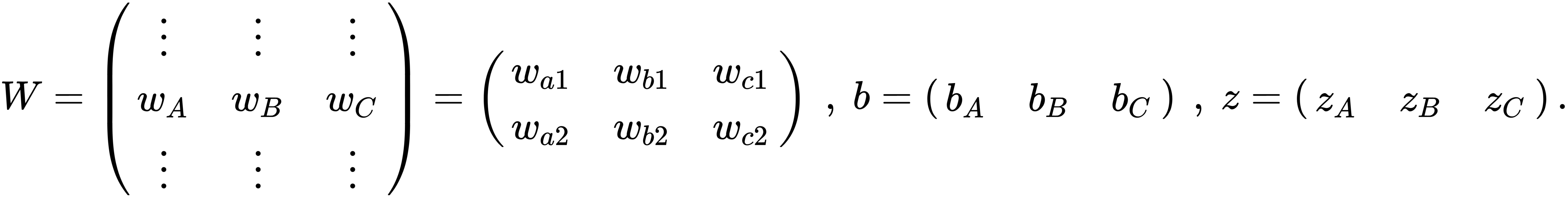The activation of the first layer can, therefore, be written as a vector,, which can be directly passed as an input vector to the next layer, and so on until the last layer.

# Implementation

Like the single neuron, this model can be implemented in Python. Actually, we do not even have to make too many edits compared to our Neuron class:

`import numpy as npclass FullyConnectedLayer(object):    """A simple fully-connected NN layer.    Args:        num_inputs (int): The input vector size/number of input values.        layer_size (int): The output vector size/number of neurons.        activation_fn (callable): The activation function for this layer.    Attributes:        W (ndarray): The weight values for each input.        b (ndarray): The bias value, added to the weighted sum.        size (int): The layer size/number of neurons.        activation_fn (callable): The neurons' activation function.    """    def __init__(self, num_inputs, layer_size, activation_fn):        super().__init__()        # Randomly initializing the parameters (using a normal distribution this time):        self.W = np.random.standard_normal((num_inputs, layer_size))        self.b = np.random.standard_normal(layer_size)        self.size = layer_size        self.activation_fn = activation_fn    def forward(self, x):        """Forward the input signal through the layer."""        z = np.dot(x, self.W) + self.b        return self.activation_fn(z)`

We just have to change the dimensionality of some of the variables in order to reflect the multiplicity of neurons inside a layer. With this implementation, our layer can even process several inputs at once! Passing a single column vector x (of shape 1 × s with s number of values in x) or a stack of column vectors (of shape n × s with n number of samples) does not change anything with regard to our matrix calculations, and our layer will correctly output the stacked results (assuming b is added to each row):

`np.random.seed(42)# Random input column-vectors of 2 values (shape = `(1, 2)`):x1 = np.random.uniform(-1, 1, 2).reshape(1, 2)# > [[-0.25091976  0.90142861]]x2 = np.random.uniform(-1, 1, 2).reshape(1, 2)    # > [[0.46398788 0.19731697]]relu_fn = lambda y: np.maximum(y, 0)    # Defining our activation functionlayer = FullyConnectedLayer(2, 3, relu_fn)# Our layer can process x1 and x2 separately...out1 = layer.forward(x1)# > [[0.28712364 0.         0.33478571]]out2 = layer.forward(x2)# > [[0.         0.         1.08175419]]# ... or together:x12 = np.concatenate((x1, x2))  # stack of input vectors, of shape `(2, 2)`out12 = layer.forward(x12)# > [[0.28712364 0.         0.33478571]#    [0.         0.         1.08175419]]`
A stack of input data is commonly called a batch.

With this implementation, it is now just a matter of chaining fully connected layers together to build simple neural networks.

# Applying our network to classification

We know how to define layers, but have yet to initialize and connect them into networks for computer vision. To demonstrate how to do this, we will tackle a famous recognition task.

Classifying images of handwritten digits (that is, recognizing whether an image contains a 0 or a 1 and so on) is a historical problem in computer vision. The Modified National Institute of Standards and Technology (MNIST) dataset (http://yann.lecun.com/exdb/mnist/), which contains 70,000 grayscale images (28 × 28 pixels) of such digits, has been used as a reference over the years so that people can test their methods for this recognition task (Yann LeCun and Corinna Cortes hold all copyrights for this dataset, which is shown in the following diagram):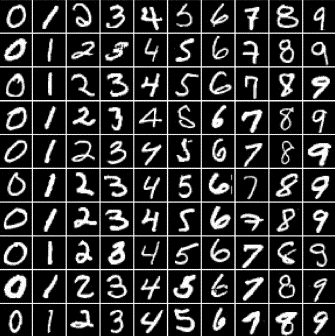Figure 1.14: Ten samples of each digit from the MNIST dataset

For digit classification, what we want is a network that takes one of these images as input and returns an output vector expressing how strongly the network believes the image corresponds to each class. The input vector has 28 × 28 = 784 values, while the output has 10 values (for the 10 different digits, from 0 to 9). In-between all of this, it is up to us to define the number of hidden layers and their sizes. To predict the class of an image, it is then just a matter of forwarding the image vector through the network, collecting the output, and returning the class with the highest belief score.

These belief scores are commonly transformed into probabilities to simplify further computations or the interpretation. For instance, let's suppose that a classification network gives a score of 9 to the class dog, and a score of 1 to the other class, cat. This is equivalent to saying that according to this network, there is a 9/10 probability that the image shows a dog and a 1/10 probability it shows a cat.

Before we implement a solution, let's prepare the data by loading the MNIST data for training and testing methods. For simplicity, we will use the mnist Python module (https://github.com/datapythonista/mnist), which was developed by Marc Garcia (under the BSD 3-Clause New or Revised license, and is already installed in this chapter's source directory):

`import numpy as npimport mnistnp.random.seed(42)# Loading the training and testing data:X_train, y_train = mnist.train_images(), mnist.train_labels()X_test,  y_test  = mnist.test_images(), mnist.test_labels()num_classes = 10    # classes are the digits from 0 to 9# We transform the images into column vectors (as inputs for our NN):X_train, X_test = X_train.reshape(-1, 28*28), X_test.reshape(-1, 28*28)# We "one-hot" the labels (as targets for our NN), for instance, transform label `4` into vector `[0, 0, 0, 0, 1, 0, 0, 0, 0, 0]`:y_train = np.eye(num_classes)[y_train]`
More detailed operations for the preprocessing and visualization of the dataset can be found in this chapter's source code.

# Implementing the network

For the neural network itself, we have to wrap the layers together and add some methods to forward through the complete network and to predict the class according to the output vector. After the layer's implementation, the following code should be self-explanatory:

`import numpy as npfrom layer import FullyConnectedLayerdef sigmoid(x): # Apply the sigmoid function to the elements of x.    return 1 / (1 + np.exp(-x)) # yclass SimpleNetwork(object):    """A simple fully-connected NN.    Args:        num_inputs (int): The input vector size / number of input values.        num_outputs (int): The output vector size.        hidden_layers_sizes (list): A list of sizes for each hidden layer to be added to the network    Attributes:        layers (list): The list of layers forming this simple network.    """    def __init__(self, num_inputs, num_outputs, hidden_layers_sizes=(64, 32)):        super().__init__()        # We build the list of layers composing the network:        sizes = [num_inputs, *hidden_layers_sizes, num_outputs]        self.layers = [            FullyConnectedLayer(sizes[i], sizes[i + 1], sigmoid)            for i in range(len(sizes) - 1)]    def forward(self, x):        """Forward the input vector `x` through the layers."""        for layer in self.layers: # from the input layer to the output one            x = layer.forward(x)        return x    def predict(self, x):        """Compute the output corresponding to `x`, and return the index of the largest output value"""        estimations = self.forward(x)        best_class = np.argmax(estimations)        return best_class    def evaluate_accuracy(self, X_val, y_val):        """Evaluate the network's accuracy on a validation dataset."""        num_corrects = 0        for i in range(len(X_val)):            if self.predict(X_val[i]) == y_val[i]:                num_corrects += 1        return num_corrects / len(X_val)`

We just implemented a feed-forward neural network that can be used for classification! It is now time to apply it to our problem:

`# Network for MNIST images, with 2 hidden layers of size 64 and 32:mnist_classifier = SimpleNetwork(X_train.shape, num_classes, [64, 32])# ... and we evaluate its accuracy on the MNIST test set:accuracy = mnist_classifier.evaluate_accuracy(X_test, y_test)print("accuracy = {:.2f}%".format(accuracy * 100))# > accuracy = 12.06%`

We only got an accuracy of ~12.06%. This may look disappointing since it is an accuracy that's barely better than random guessing. But it makes sense—right now, our network is defined by random parameters. We need to train it according to our use case, which is a task that we will tackle in the next section.

# Training a neural network

Neural networks are a particular kind of algorithm because they need to be trained, that is, their parameters need to be optimized for a specific task by making them learn from available data. Once the networks are optimized to perform well on this training dataset, they can be used on new, similar data to provide satisfying results (if the training was done properly).

Before solving the problem of our MNIST task, we will provide some theoretical background, cover different learning strategies, and present how training is actually done. Then, we will directly apply some of these notions to our example so that our simple network finally learns how to solve the recognition task!

# Learning strategies

When it comes to teaching neural networks, there are three main paradigms, depending on the task and the availability of training data.

# Supervised learning

Supervised learning may be the most common paradigm, and it is certainly the easiest to grasp. It applies when we want to teach neural networks a mapping between two modalities (for example, mapping images to their class labels or to their semantic masks). It requires access to a training dataset containing both the images and their ground truth labels (such as the class information per image or the semantic masks).

With this, the training is then straightforward:

• Give the images to the network and collect its results (that is, predicted labels).
• Evaluate the network's loss, that is, how wrong its predictions are when comparing it to the ground truth labels.
• Adjust the network parameters accordingly to reduce this loss.
• Repeat until the network converges, that is, until it cannot improve further on this training data.

Therefore, this strategy deserves the adjective supervised—an entity (us) supervises the training of the network by providing it with feedback for each prediction (the loss computed from the ground truths) so that the method can learn by repetition (it was correct/false; try again).

# Unsupervised learning

However, how do we train a network when we do not have any ground truth information available? Unsupervised learning is one answer to this. The idea here is to craft a function that computes the network's loss only based on its input and its corresponding output.

This strategy applies very well to applications such as clustering (grouping images with similar properties together) or compression (reducing the content size while preserving some properties). For clustering, the loss function could measure how similar images from one cluster are compared to images from other clusters. For compression, the loss function could measure how well preserved the important properties are in the compressed data compared to the original ones.

Unsupervised learning thus requires some expertise regarding the use cases so that we can come up with meaningful loss functions.

# Reinforcement learning

Reinforcement learning is an interactive strategy. An agent navigates through an environment (for example, a robot moving around a room or a video game character going through a level). The agent has a predefined list of actions it can make (walk, turn, jump, and so on) and, after each action, it ends up in a new state. Some states can bring rewards, which are immediate or delayed, and positive or negative (for instance, a positive reward when the video game character touches a bonus item, and a negative reward when it is hit by an enemy).

At each instant, the neural network is provided only with observations from the environment (for example, the robot's visual feed, or the video game screen) and reward feedback (the carrot and stick). From this, it has to learn what brings higher rewards and estimate the best short-term or long-term policy for the agent accordingly. In other words, it has to estimate the series of actions that would maximize its end reward.

Reinforcement learning is a powerful paradigm, but it is less commonly applied to computer vision use cases. It won't be presented further here, though we encourage machine learning enthusiasts to learn more.

# Teaching time

Whatever the learning strategy, the overall training steps are the same. Given some training data, the network makes its predictions and receives some feedback (such as the results of a loss function), which is then used to update the network's parameters. These steps are then repeated until the network cannot be optimized further. In this section, we will detail and implement this process, from loss computation to weights optimization.

# Evaluating the loss

The goal of the loss function is to evaluate how well the network, with its current weights, is performing. More formally, this function expresses the quality of the predictions as a function of the network's parameters (such as its weights and biases). The smaller the loss, the better the parameters are for the chosen task.

Since loss functions represent the goal of networks (return the correct labels, compress the image while preserving the content, and so on), there are as many different functions as there are tasks. Still, some loss functions are more commonly used than others. This is the case for the sum-of-squares function, also called L2 loss (based on the L2 norm), which is omnipresent in supervised learning. This function simply computes the squared difference between each element of the output vector y (the per-class probabilities estimated by our network) and each element of the ground truth vector ytrue (the target vector with null values for every class but the correct one):There are plenty of other losses with different properties, such as L1 loss, which computes the absolute difference between the vectors, or binary cross-entropy (BCE) loss, which converts the predicted probabilities into a logarithmic scale before comparing them to the expected values:The logarithmic operation converts the probabilities from [0, 1] into [-, 0]. So, by multiplying the results by -1, the loss value moves from +to 0 as the neural network learns to predict properly. Note that the cross-entropy function can also be applied to multi-class problems (not just binary).

It is also common for people to divide the losses by the number of elements in the vectors, that is, computing the mean instead of the sum. The mean square error (MSE) is the averaged version of the L2 loss, and the mean absolute error (MAE) is the average version of the L1 loss.

For now, we will stick with the L2 loss as an example. We will use it for the rest of the theoretical explanations, as well as to train our MNIST classifier.

# Backpropagating the loss

How can we update the network parameters so that they minimize the loss? For each parameter, what we need to know is how slightly changing its value would affect the loss. If we know which changes would slightly decrease the loss, then it is just a matter of applying these changes and repeating the process until reaching a minimum. This is exactly what the gradient of the loss function expresses, and what the gradient descent process is.

At each training iteration, the derivatives of the loss with respect to each parameter of the network are computed. These derivatives indicate which small changes to the parameters need to be applied (with a -1 coefficient since the gradient indicates the direction of increase of the function, while we want to minimize it). It can be seen as walking step by step down the slope of the loss function with respect to each parameter, hence the name gradient descent for this iterative process (refer to the following diagram):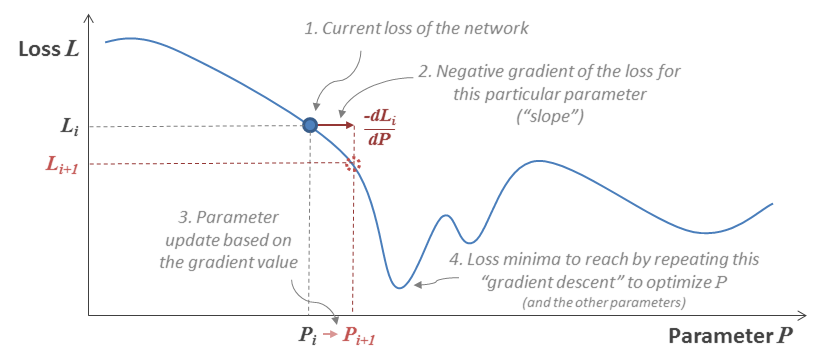Figure 1.15: Illustrating the gradient descent to optimize a parameter P of the neural network

The question now is, how can we compute all of these derivatives (the slope values as a function of each parameter)? This is where the chain rule comes to our aid. Without going too deep into calculus, the chain rule tells us that the derivatives with respect to the parameters of a layer, k, can be simply computed with the input and output values of that layer (xk, yk), and the derivatives of the following layer, k + 1. More formally, for the layer's weights, Wk, we have the following:Here, l'k+1 is the derivative that is computed for layer k + 1 with respect to its input, xk+1 = yk, with f'k being the derivative of the layer's activation function, andbeing the transpose of x. Note that zk represents the result of the weighted sum performed by the layer k (that is, before the input of the layer's activation function), as defined in the Layering neurons together section. Finally, thesymbol represents the element-wise multiplication between two vectors/matrices. It is also known as the Hadamard product. As shown in the following equation, it basically consists of multiplying the elements pair-wise:Back to the chain rule, the derivatives with respect to the bias can be computed in a similar fashion, as follows:Finally, to be exhaustive, we have the following equation:These calculations may look complex, but we only need to understand what they represent—we can compute how each parameter affects the loss recursively, layer by layer, going backward (using the derivatives for a layer to compute the derivatives for the previous layer). This concept can also be illustrated by representing neural networks as computational graphs, that is, as graphs of mathematical operations chained together (the weighted summation of the first layer is performed and its result is passed to the first activation function, then its own output is passed to the operations of the second layer, and so on). Therefore, computing the result of a whole neural network with respect to some inputs consists of forwarding the data through this computational graph, while obtaining the derivatives with respect to each of its parameters consists of propagating the resulting loss through the graph backward, hence the term backpropagation.

To start this process by the output layer, the derivatives of the loss itself with respect to the output values are needed (refer to the previous equation). Therefore, it is primordial that the loss function can be easily derived. For instance, the derivative of the L2 loss is simply the following:As we mentioned earlier, once we know the loss derivatives with respect to each parameter, it is just a matter of updating them accordingly:As we can see, the derivatives are often multiplied by a factor(epsilon) before being used to update the parameters. This factor is called the learning rate. It helps to control how strongly each parameter should be updated at each iteration. A large learning rate may allow the network to learn faster, but with the risk of making steps so big that the network may miss the loss minimum. Therefore, its value should be set with care. Let's now summarize the complete training process:

1. Select the n next training images and feed them to the network.
2. Compute and backpropagate the loss, using the chain rule to get the derivatives with respect to the parameters of the layers.
3. Update the parameters with the values of the corresponding derivatives (scaled with the learning rate).
4. Repeat steps 1 to 3 to iterate over the whole training set.
5. Repeat steps 1 to 4 until convergence or until a fixed number of iterations.

One iteration over the whole training set (steps 1 to 4) is called an epoch. If n = 1 and the training sample is randomly selected among the remaining images, this process is called stochastic gradient descent (SGD), which is easy to implement and visualize, but slower (more updates are done) and noisier. People tend to prefer mini-batch stochastic gradient descent. It implies using larger n values (limited by the capabilities of the computer) so that the gradient is averaged over each mini-batch (or, more simply, named batch) of n random training samples (and is thus less noisy).

Nowadays, the term SGD is commonly used, regardless of the value of n.

In this section, we have covered how neural networks are trained. It is now time to put this into practice!

# Teaching our network to classify

So far, we have only implemented the feed-forward functionality for our network and its layers. First, let's update our FullyConnectedLayer class so that we can add methods for backpropagation and optimization:

`class FullyConnectedLayer(object):    # [...] (code unchanged)    def __init__(self, num_inputs, layer_size, activation_fn, d_activation_fn):        # [...] (code unchanged)        self.d_activation_fn = d_activation_fn # Deriv. activation function         self.x, self.y, self.dL_dW, self.dL_db = 0, 0, 0, 0 # Storage attr.    def forward(self, x):        z = np.dot(x, self.W) + self.b        self.y = self.activation_fn(z)        self.x = x  # we store values for back-propagation        return self.y    def backward(self, dL_dy):        """Back-propagate the loss."""        dy_dz = self.d_activation_fn(self.y)  # = f'        dL_dz = (dL_dy * dy_dz) # dL/dz = dL/dy * dy/dz = l'_{k+1} * f'        dz_dw = self.x.T        dz_dx = self.W.T        dz_db = np.ones(dL_dy.shape) # dz/db = "ones"-vector        # Computing and storing dL w.r.t. the layer's parameters:        self.dL_dW = np.dot(dz_dw, dL_dz)        self.dL_db = np.dot(dz_db, dL_dz)        # Computing the derivative w.r.t. x for the previous layers:        dL_dx = np.dot(dL_dz, dz_dx)        return dL_dx    def optimize(self, epsilon):        """Optimize the layer's parameters w.r.t. the derivative values."""        self.W -= epsilon * self.dL_dW        self.b -= epsilon * self.dL_db`
The code presented in this section has been simplified and stripped of comments to keep its length reasonable. The complete sources are available in this book's GitHub repository, along with a Jupyter notebook that connects everything together.

Now, we need to update the SimpleNetwork class by adding methods to backpropagate and optimize layer by layer, and a final method to cover the complete training:

`def derivated_sigmoid(y):  # sigmoid derivative function    return y * (1 - y)def loss_L2(pred, target): # L2 loss function    return np.sum(np.square(pred - target)) / pred.shape # opt. for results not depending on the batch size (pred.shape), we divide the loss by itdef derivated_loss_L2(pred, target):    # L2 derivative function    return 2 * (pred - target) # we could add the batch size division here too, but it wouldn't really affect the training (just scaling down the derivatives).class SimpleNetwork(object): # [...] (code unchanged) def __init__(self, num_inputs, num_outputs, hidden_layers_sizes=(64, 32), loss_fn=loss_L2, d_loss_fn=derivated_loss_L2):        # [...] (code unchanged, except for FC layers new params.)        self.loss_fn, self.d_loss_fn = loss_fn, d_loss_fn    # [...] (code unchanged)    def backward(self, dL_dy):        """Back-propagate the loss derivative from last to 1st layer."""        for layer in reversed(self.layers):            dL_dy = layer.backward(dL_dy)        return dL_dy def optimize(self, epsilon):        """Optimize the parameters according to the stored gradients."""        for layer in self.layers:            layer.optimize(epsilon)    def train(self, X_train, y_train, X_val, y_val, batch_size=32, num_epochs=5, learning_rate=5e-3):        """Train (and evaluate) the network on the provided dataset."""        num_batches_per_epoch = len(X_train) // batch_size        loss, accuracy = [], []        for i in range(num_epochs): # for each training epoch            epoch_loss = 0            for b in range(num_batches_per_epoch): # for each batch                # Get batch:                b_idx = b * batch_size                b_idx_e = b_idx + batch_size                x, y_true = X_train[b_idx:b_idx_e], y_train[b_idx:b_idx_e]                # Optimize on batch:                y = self.forward(x) # forward pass                epoch_loss += self.loss_fn(y, y_true) # loss                 dL_dy = self.d_loss_fn(y, y_true) # loss derivation                self.backward(dL_dy) # back-propagation pass                self.optimize(learning_rate) # optimization                        loss.append(epoch_loss / num_batches_per_epoch)            # After each epoch, we "validate" our network, i.e., we measure its accuracy over the test/validation set:            accuracy.append(self.evaluate_accuracy(X_val, y_val))            print("Epoch {:4d}: training loss = {:.6f} | val accuracy = {:.2f}%".format(i, loss[i], accuracy[i] * 100))`

Everything is now ready! We can train our model and see how it performs:

```losses, accuracies = mnist_classifier.train(    X_train, y_train, X_test, y_test, batch_size=30, num_epochs=500)# > Epoch    0: training loss = 1.096978 | val accuracy = 19.10%
# > Epoch    1: training loss = 0.886127 | val accuracy = 32.17%
# > Epoch    2: training loss = 0.785361 | val accuracy = 44.06%# [...]# > Epoch  498: training loss = 0.046022 | val accuracy = 94.83%
# > Epoch  499: training loss = 0.045963 | val accuracy = 94.83%```

Congratulations! If your machine is powerful enough to complete this training (this simple implementation does not take advantage of the GPU), we just obtained our very own neural network that is able to classify handwritten digits with an accuracy of ~94.8%!

# Training considerations – underfitting and overfitting

We invite you to play around with the framework we just implemented, trying different hyperparameters (layer sizes, learning rate, batch size, and so on). Choosing the proper topography (as well as other hyperparameters) can require lots of tweaking and testing. While the sizes of the input and output layers are conditioned by the use case (for example, for classification, the input size would be the number of pixel values in the images, and the output size would be the number of classes to predict from), the hidden layers should be carefully engineered.

For instance, if the network has too few layers, or the layers are too small, the accuracy may stagnate. This means the network is underfitting, that is, it does not have enough parameters for the complexity of the task. In this case, the only solution is to adopt a new architecture that is more suited to the application.

On the other hand, if the network is too complex and/or the training dataset is too small, the network may start overfitting the training data. This means that the network will learn to fit very well to the training distribution (that is, its particular noise, details, and so on), but won't generalize to new samples (since these new images may have a slightly different noise, for instance). The following diagram highlights the differences between these two problems. The regression method on the extreme left does not have enough parameters to model the data variations, while the method on the extreme right has too many, which means it will struggle to generalize: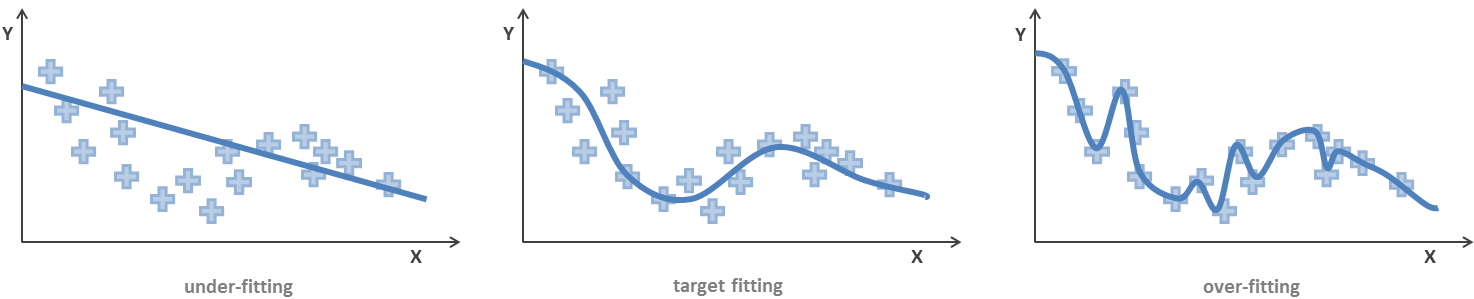Figure 1.16: A common illustration of underfitting and overfitting

While gathering a larger, more diverse training dataset seems the logical solution to overfitting, it is not always possible in practice (for example, due to limited access to the target objects). Another solution is to adapt the network or its training in order to constrain how much detail the network learns. Such methods will be detailed in Chapter 3, Modern Neural Networks, among other advanced neural network solutions.

# Summary

We covered a lot of ground in this first chapter. We introduced computer vision, the challenges associated with it, and some historical methods, such as SIFT and SVMs. We got familiar with neural networks and saw how they are built, trained, and applied. After implementing our own classifier network from scratch, we can now better understand and appreciate how machine learning frameworks work.

With this knowledge, we are now more than ready to start with TensorFlow in the next chapter.

# Questions

1. Which of the following tasks does not belong to computer vision?
• A web search for images similar to a query
• A 3D scene reconstruction from image sequences
• Animation of a video character
2. Which activation function were the original perceptrons using?
3. Suppose we want to train a method to detect whether a handwritten digit is a 4 or not. How should we adapt the network that we implemented in this chapter for this task?

• ##### Benjamin Planche

Benjamin Planche is a passionate PhD student at the University of Passau and Siemens Corporate Technology. He has been working in various research labs around the world (LIRIS in France, Mitsubishi Electric in Japan, and Siemens in Germany) in the fields of computer vision and deep learning for more than five years. Benjamin has a double master's degree with first-class honors from INSA-Lyon, France, and the University of Passau, Germany. His research efforts are focused on developing smarter visual systems with less data, targeting industrial applications. Benjamin also shares his knowledge and experience on online platforms, such as StackOverflow, or applies this knowledge to the creation of aesthetic demonstrations.

Browse publications by this author
• ##### Eliot Andres

Eliot Andres is a freelance deep learning and computer vision engineer. He has more than 3 years' experience in the field, applying his skills to a variety of industries, such as banking, health, social media, and video streaming. Eliot has a double master's degree from École des Ponts and Télécom, Paris. His focus is industrialization: delivering value by applying new technologies to business problems. Eliot keeps his knowledge up to date by publishing articles on his blog and by building prototypes using the latest technologies.

Browse publications by this author

### Latest Reviews

(1 reviews total)